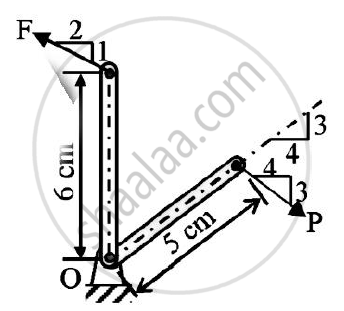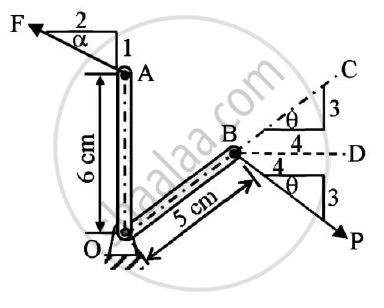# In the Rocket Arm Shown in the Figure the Moment of ‘F’ About ‘O’ Balances that P=250 N.Find F. - Engineering Mechanics

In the rocket arm shown in the figure the moment of ‘F’ about ‘O’ balances that P=250 N.Find F.#### Solution

Solution :
Given : P = 250 N
To find : Magnitude of force F
Solution :tan alpha =1/2
=0.5
alpha =26.5651°

tan θ (DE)/(AD)=(DE)/(BC)=3/4=0.75

θ = 36.87°

∠CBD = ∠PBD = θ = 36.87°
∠CBP=2 θ = 2 x 36.87 = 73.74°
It is given that at O the moment of F about O balances the moment of P
Fcos α x OA = Psin2 θ x OB
Fcos26.5651 x 6 = 250sin 73.74 x 5
F=223.6068 N
Magnitude of force F= 223.6068 N

Concept: Resultant of concurrent forces
Is there an error in this question or solution?